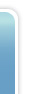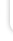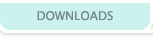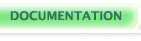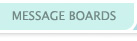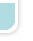## Operators

For those of you familiar with C, I'll give you a quick rundown (everyone else skip this paragraph): VERGE has pretty much all the C operators you'll need except pointer stuff. It has no order of operations. There's no *=, /=, or %=, but there is a += and -=. The assignment operators do not return values and as such cannot be used inside an arithmetic or logical expression. Since all integers are signed, bitshifting is automatically signed as well.

Verge contains five kinds of "operators" used to build statements. An operator is a symbol which, when combined with one or more "operands" (constants, variables, or expressions), changes the value of the operands or produces a new value based on them. For example, the + arithmetic operator takes two integer operands and produces a new integer which is the sum of those two.

Operators are either unary or binary; that is, they take either one or two operands. Unary operators either precede or follow their operands, whereas binary operators have are placed in between their two operands. The + operator mentioned above is binary, so its operators are placed on either side; to produce the sum of 5 and 6, we type "5 + 6". Except where otherwise stated, all the operators described below are binary.

ARITHMETIC OPERATORS:

The arithmetic operators represent fundamental mathematical operations such as addition and subtraction.

Addition (+): The addition operator generates a new number that is the sum of its operands.

Subtraction (-): The subtraction operator generates a new number that is the difference of its operands. Order is important; the operand after the minus sign is subtracted from the one before it.

Multiplication (*): The multiplication operator generates a new number that is the product of its operands.

Division (/): The division operator generates a new number that is the quotient of its operands. As with subtraction, order is important; the first operand is the numerator, the second the denominator. This is integer division, which means that the final value is always an integer, rounded down if necessary.

Modulus (%): The modulus operator returns the remainder of the division of the first operand by the second.

Arithmetic operators can be combined into complex arithmetic expressions. They are evaluated from left to right; that is to say, there is no order of operations. To enforce a specific evaluation order, you may use parentheses.

```// Arithmetic Operator examples
int n = 0;      // n starts out as 0

n = 2 + 3;      // Addition: n is now 5
n = 12 - n;     // Subtraction: n is now 7
n = 2 * 4;      // Multiplication: n is now 8
n = n / 2;      // Division: n is now 4
n = 10 % 3;     // Modulus: n is now 1 (the remainder of 10 / 3)

// Examples of complex expressions

n = 1 + 3 * 5;                    // n is now 20 (since there's no order of operations)
n = 100 % (9 * 3);                // n is now 19 (the remainder of 100 / 27)
n = 2 * (5 - (7 * 3));            // n is now -32

```

BITWISE OPERATORS:

Bitwise operators are so called because they operate individually on every bit of a (32-bit signed) integer. Because they manipulate numbers on a binary level, they are somewhat tricky for beginners. If you feel comfortable with them, however, you can use bitwise operators freely in arithmetic expressions.

Bitwise OR (|): The Bitwise OR generates a new value by ORing each bit of the two operands. What this means is that the new value will have a 1 in each bit location where either or both of the operands had a 1, and a 0 in every other bit location.

Bitwise AND (&): The Bitwise AND generates a new value by ANDing each bit of the two operands. What this means is that the new value will have a 0 in each bit location where either or both of the operands had a 0, and a 1 in every other bit location.

Bitwise XOR (^): The Bitwise XOR generates a new value by exclusive-ORing each bit of the two operands. What this means is that the new value will have a 1 in each bit location where either one or the other but not both of the operands had a 1, and a 0 in every other bit location.

Bitwise NOT (~): The Bitwise NOT, a unary operator that precedes its operand, generates a new value by inverting each bit in the bitfield. All bits that were 1 are now 0, and all bits that were 0 are now 1. To toggle a single bit, use a bitwise XOR of the notted bit

Left Bitshift (<<): The Left Bitshift operator generates a new number which is its first operand with all the bits shifted left a number of spaces equal to the value of the second operand. If this sounds confusing, here's an easier way to think about it: in the expression m << n, the value returned is equal to m multiplied by 2 to the nth power.

Right Bitshift (>>): The Right Bitshift operator generates a new number which its its first operand with all the bits shifted right a number of spaces equal to the value of the second operand. In the expression m>>n, the value returned is equal to m divided by 2 to the nth power (using integer division, naturally).

LOGICAL OPERATORS:

The logical operators can be used only in the conditions of if and while statements. They return either true (a nonzero number) or false (zero). Like arithmetic operators, logical operators take integer variables, constants, or expressions (arithmetic or logical) as their operands, and are evaluated from left to right.

Equality (== or =): The Equality operator returns true if the two operands are equal; otherwise, it returns false. It is often represented as a double equals sign to differentiate it from the Assignment operator, but this distinction isn't necessary. Note that neither strings nor structs can be used as logical operands, so this operator cannot be used to test their equality.

Inequality (!=): The Inequality operator returns true if the two operands are inequal; otherwise, it returns false.

Greater Than (>): Greater Than returns true if the first operand is greater than the second; otherwise, it returns false.

Lesser Than (<): Lesser Than returns true if the first operand is lesser than the second; otherwise, it returns false.

Greater Than or Equal To (>=): Greater Than or Equal To returns true if the first operand is greater than or equal to the second; otherwise, it returns false.

Lesser Than or Equal To (<=): Lesser Than or Equal To returns true if the first operand is lesser than or equal to the second; otherwise, it returns false.

Logical OR (||) (also simply the keyword "or"): The logical OR returns true if either or both of its operands are true; otherwise, it returns false.

Logical AND (&&) (also simply the keyword "and"): The logical OR returns true only if both of its operands are true; otherwise, it returns false.

Logical NOT (!) (also simply the keyword "not"): The logical NOT is a unary operator that precedes its operand. It returns true if its operand is false; otherwise it returns true.

Note that there is no logical XOR in VERGE.

ASSIGNMENT OPERATORS:

Assignment operators are special in that they actually change the value of one of their operands. The operand to be changed must be a variable, since only variables can have their values altered. Assignment operators are also unique because they do not return values; for this reason, they cannot be used inside expressions.

Assignment (=): The ordinary assignment operator changes the the value of the first operand to the value of the second.

Increment (++): Increment is a unary operator that follows its operand. It increases the value of its single operand by 1.

Decrement (--): Decrement is a unary operator that follows its operand. It decreases the value of its single operand by 1.

Increase (+=): The Increase operator increases the value of the first operand by the value of the second.

Decrease (-=): The Decrease operator decreases the value of the first operand by the value of the second.

The ordinary assignment operator (=) can be used within a declaration statement to initialize a newly declared variable to a specific value.

```// Assignment operator examples
int n = 2;        // n is declared and assigned the value of 2
n = 7;            // n is assigned the value of 7
n++;              // n is now 8
n--;              // n is now 7 again
n += 12 + n;      // n is now 26
n -= n;           // n is now 0
```

STRING OPERATORS:

There are only two string operators in VERGE. They accept only string variables, string literals, and functions that return strings as their operands.

String Concatenation (+): The string concatenation operator is the plus sign, just like the addition operator. Since the operator is preceded by its first operand, the compiler figures out whether it's doing addition or concatenation by looking at the type (int or string) of that operand. Concatenation produces a new string composed of the second operand appended to the first.

String Assignment (=): The String Assignment operator is identical to the ordinary assignment operator, except it functions on strings rather than integers.

```// String concatenation examples
string s = "";                     // s is declared and set to an empty string
string s = "hell" + "o";           // s is now "hello"
string s = "why " + s + " there";  // s is now "why hello there"
```

As of Verge 3.1, the += operator is a valid shortcut to add strings together.

```string s = "Fish";
s = s + " and Chips"; // The old way of joining strings together
s += " and Chips"; // Now a valid shortcut.```

As of Verge 3.1, you can put parentheses directly after a string, and the compiler will interpret that as a CallFunction.

```CallFunction("myfunction"); // Uses CallFunction call the function named "myfunction" at runtime.
"myfunction"(); // Same thing, but less typing, and can make string variables look more like function pointers in some code.
```
Talkback

Post a new comment?

Talkback #4 written by Feyr on 2004-10-28.

There's an error in the left bitshift operator docs. The last sentence should read: In the expression m<<n, the value returned is equal to m multiplied by 2 to the nth power. rather than being truncated at the < symbol.

Talkback #3 written by rpgking on 2004-08-25.

Here's how the XOR bitwise operator can be used for a swap function that uses no temp variable: [PSEUDOCODE]

```Swap(int a, int b)
{
a = a  XOR b;
b = a  XOR b;
a = a  XOR b;
}
```
[VERGE C]
```//general swap function for two integers (bitwise)
void SwapInt(string int_a, string int_b)
{
SetInt(int_a, GetInt(int_a)^GetInt(int_b));
SetInt(int_b, GetInt(int_a)^GetInt(int_b));
SetInt(int_a, GetInt(int_a)^GetInt(int_b));
}

//can swap two values of a single array or between two different arrays (bitwise)
void SwapArrayValues(string array_a, int index_a, string array_b, int index_b)
{
SetIntArray(array_a, index_a, GetIntArray(array_a, index_a) ^ GetIntArray(array_b, index_b));
SetIntArray(array_b, index_b, GetIntArray(array_a, index_a) ^ GetIntArray(array_b, index_b));
SetIntArray(array_a, index_a, GetIntArray(array_a, index_a) ^ GetIntArray(array_b, index_b));
}
```
Both of these functions have been tested and work in VERGE 3. -rpgking

Talkback #2 written by mcgrue on 2004-08-01.

This division truncation Gannon refers to is known formally in computer science circles as "Integer Division". When dealing explicitly with integers, there can be no fractional results. As of this writing, there's no native support for floating-point division (ie, numbers with decimal points in them) in v3. However, aen has crafted a short tutorial in this very manual about [fixed-point math], which explains how to sneak around these rules and get some fractional math out of integers.

Talkback #1 written by gannon on 2004-08-01.

one thing about Division it doesn't round down exactly it truncates (gets rid of everything after the .) ie. n = 4/5 //n is 0 n = 6/5 //n is 1 n = (0-6)/5 //n is -1(just rounding down would give you -2)

Post a new comment?

 Doc Nav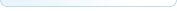Your docs View All Docs If you log in, you can edit the documentation, or create your own documents and tutorials!Ben McGraw's lovingly crafted this website from scratch for years.
It's a lot prettier this go around because of Jon Wofford.
Verge-rpg.com is a member of the lunarnet irc network, and would like to take this opportunity to remind you that regardless how babies taste, it is wrong to eat them.